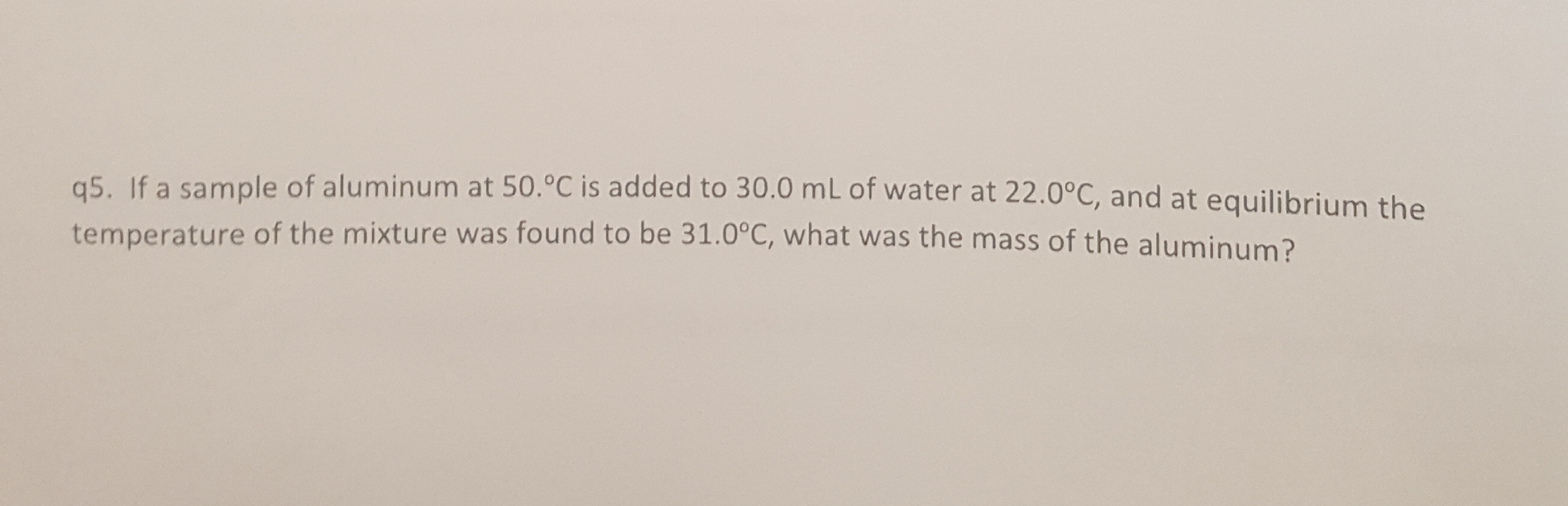q5. If a sample of aluminum at 50.°C is added to 30.0 mL of water at 22.0°c, and at equilibrium thtemperature of the mixture was found to be 31.0°C, what was the mass of the aluminum?the

Questionhelp_outlineImage Transcriptioncloseq5. If a sample of aluminum at 50.°C is added to 30.0 mL of water at 22.0°c, and at equilibrium th temperature of the mixture was found to be 31.0°C, what was the mass of the aluminum? the fullscreen
Step 1

Mass of the aluminum is to be calculated when the following data is given-

Initial Temperature of the aluminum, Ti,Al = 50.0 º C

Final temperature of the mixture, Tf = 31.0 ºC

Volume of water = 30.0 mL

Initial temperature of water, Ti,w = 22.0º C

Step 2

This is a thermo- equilibrium situation. At the point of equilibrium, heat lost by the metal would be equal to the heat gained by the water.

Step 3

To calculate the heat gained by water, first determi...

Want to see the full answer?

See Solution

Want to see this answer and more?

Our solutions are written by experts, many with advanced degrees, and available 24/7

See Solution
Tagged in

Physical Chemistry